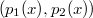﻿

### Existence and multiplicity results for Dirichlet boundary value problems involving the$(p_{1}(x),p_{2}(x))$- Laplace operator

#### Abstract

This paper is concerned with the existence and multiplicity of solutions for the following Dirichlet boundary value problems involving the$(p_{1}(x),p_{2}(x))$-Laplace operator of the form: \begin{equation*} \begin{gathered} -\operatorname{div}(|\nabla u|^{p_{1}(x)-2}\nabla u)- \operatorname{div}(|\nabla u|^{p_{2}(x)-2}\nabla u)= f(x,u) \quad\text{in } \Omega,\\ u=0 \quad \text{on } \partial\Omega. \end{gathered} \end{equation*} By means of critical point theorems with Cerami condition and the theory of the variable exponent Sobolev spaces, we establish the existence and multiplicity of solutions.

DOI Code: 10.1285/i15900932v37n1p69

Keywords: variational methods; generalized Lebesgue-Sobolev spaces

Full Text: PDF